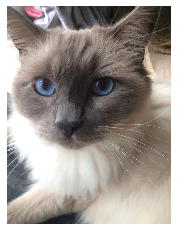# Handy commands for manipulating images in Python

Posted on Tue 03 September 2019 in Python

In this post I show some handy commands for working with image data in Python. The following packages are useful when working with image data.

In :
import cv2
import glob
import numpy as np
import matplotlib.pyplot as plt


## 1. Read multiple image files in a directory¶

In :
images = [cv2.imread(file) for file in glob.glob("images/*.jpg")]


## 2. Plot image¶

We can use the matplotlib package to display our images. Note: OpenCV using BGR as it's default colour order for images whereas matplotlib uses RGB. Therefore when displaying a image loaded with OpenCV in matplotlib, the channels will be back to front. The simplest fix is to the convert the image back to RGB which can be done as follows.

In :
rgb_image = cv2.cvtColor(images, cv2.COLOR_BGR2RGB)
plt.imshow(rgb_image)
plt.axis('off')

Out:
(-0.5, 1199.5, 1599.5, -0.5)## 2. Resize images¶

Suppose we want to pass the images loaded above through a machine learning model that we've created. We'll need to scale the images so that they have the same input dimensions expected by our model. Let's suppose, our model requires images to have dimension (160, 120). We can reshape our images using the opencv package as follows.

Check existing shapes

In :
[i.shape for i in images]

Out:
[(1600, 1200, 3), (1600, 1200, 3), (4032, 3024, 3)]

We can see the dimension of the first loaded image is 1600 x 1200 x 3. The first two indices represent the y and x pixel position, and the third represents the RGB pixel value.

The following OpenCV command resizes the image to the dimensions specified in the dize argument. There isn't a best interpolation method to use for all situations. You can read about the options here.

In :
reshaped_img = cv2.resize(images, dsize=(120, 160), interpolation=cv2.INTER_CUBIC)


Check output shape is correct

In :
reshaped_img.shape

Out:
(160, 120, 3)

## 3. Flatten an image to a single dimension¶

Now we've reshaped our image to have the correct dimensions. Before we can pass our image through a machine learning model however we need to flatten out the the three colour channels of the image into a single vector.

So, for the cat image we reshaped above, we'd like to reshape it to have dimension (1, 160 * 120 * 3). This can be easily achieved by the following one liner in numpy:

In :
flat_im = reshaped_img.reshape(1, -1)

In :
flat_im.shape

Out:
(1, 57600)

The -1 in the reshape command is useful as it allows you to unpack any remaining dimensions into a single dimension